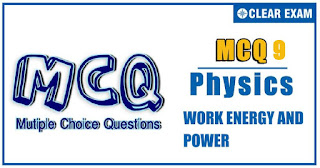## WORK ENERGY AND POWER Quiz-9

Work power energy is the most important chapter when it comes to mechanics, for JEE (Advanced). The chapter is quite tricky and takes a lot of time and devotion on your part to understand and master. But this chapter is a complete gold medal for almost all the questions of the mechanics can be solved by the work power energy approach if you master this topic..

•  a,c and d are points of equilibrium
•  a is a point of stable equilibrium
•  b is a stable equilibrium point
•  All of the above
Solution
For equilibrium, potential energy has to be minimum, maximum or constant

Q3.
A particle moves along a circle with its speed increasing at a constant rate. Choose the incorrect statement
•  Net force acting on the particle is towards the centre of the circle
•  The centripetal force does no work on the particle
•  Net work is being done on the particle
•  None of the above
Solution
Since the speed of the particle increase, it kinetic energy also increases(1/2 mv^2 ). So, work is being done on the particle. However, since centripetal force is normal to the direction of displacement, no work is done by the centripetal force

Q4.
A ball hits the floor and rebounds after inelastic collision. In this case
•  The momentum of the ball just after the collision is the same as that just before the collision
•  The mechanical energy of the ball remains the same in the collision
•  The total momentum of the ball and the earth is conserved
•  The total energy of the ball and the earth is conserved
Solution
By the conservation of momentum in the absence of external force total momentum of the system (ball + earth) remains constant

•  g/2
•  √g
•  g
•  None possible
Q6.
The kinetic energy acquired by a mass m in travelling a certain distance d, starting from rest, under the action of a force F such that the force F is directly proportional to t is
•   Directly proportional to t^2
•   Independent of t
• Directly proportional to t^4
•  Directly proportional to t
Q7.
When a mass of 2 kg is suspended slowly by a spring with its upper end fixed to the ceiling, the spring stretches by 1 cm. work done by the spring is (g=10 ms^(-2))
•  -0.2 J
•  -0.1 J
•  -0.4 J
•  None of these
Q8.
When a bicycle accelerated on a leveled road,
•  The net work done by the friction is positive
•  The net work done by the friction is zero
•  The friction on the front wheel does positive work
•  The friction on the rear wheel does negative work
•  (√3/2) √g
•  (√3/4) √g
•  √g/(2√3)
•  √g/2
Q10.
A particle is projected at an angle θ=30° with the horizontal. Which of the following curves best represents the variation of KE and potential energy as a function of time? (take the point of projection as the reference level for the gravitational potential energy)#### Written by: AUTHORNAME

AUTHORDESCRIPTION## Want to know more

Please fill in the details below:

## Latest NEET Articles\$type=three\$c=3\$author=hide\$comment=hide\$rm=hide\$date=hide\$snippet=hide

Name

ltr
item
BEST NEET COACHING CENTER | BEST IIT JEE COACHING INSTITUTE | BEST NEET, IIT JEE COACHING INSTITUTE: Work Energy and Power-Quiz-9
Work Energy and Power-Quiz-9
https://1.bp.blogspot.com/-SWFRliuT8_0/YObs_FqWLPI/AAAAAAAAUlc/BwrY8fYjczMhYeeDXPiXhC-n84siL6oNACLcBGAsYHQ/s320/Quiz%2BImage%2B20%2B%25289%2529.jpg
https://1.bp.blogspot.com/-SWFRliuT8_0/YObs_FqWLPI/AAAAAAAAUlc/BwrY8fYjczMhYeeDXPiXhC-n84siL6oNACLcBGAsYHQ/s72-c/Quiz%2BImage%2B20%2B%25289%2529.jpg
BEST NEET COACHING CENTER | BEST IIT JEE COACHING INSTITUTE | BEST NEET, IIT JEE COACHING INSTITUTE
https://www.cleariitmedical.com/2021/07/work-energy-and-power-quiz-9.html
https://www.cleariitmedical.com/
https://www.cleariitmedical.com/
https://www.cleariitmedical.com/2021/07/work-energy-and-power-quiz-9.html
true
7783647550433378923
UTF-8